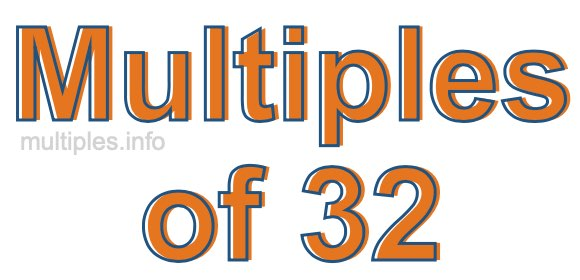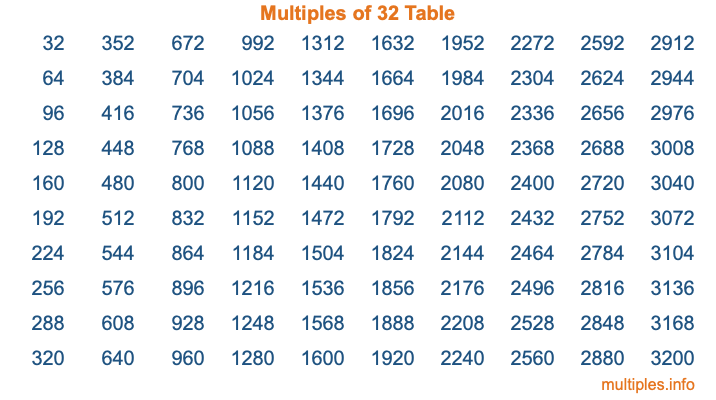Multiples of 32Welcome to the Multiples of 32 page. Here we will first teach you everything you will ever need to know about the multiples of 32, and then give you a study guide summary of everything we taught you to make sure you remember it all. Use this page to look up facts and learn information about the multiples of 32. This page will make you a multiples of thirty-two expert!

Definition of Multiples of 32
Multiples of 32 are all the numbers that when divided by 32 equal an integer. Each of the multiples of 32 are called a multiple. A multiple of 32 is created by multiplying 32 by an integer.

Therefore, to create a list of multiples of 32, you start with 1 multiplied by 32, then 2 multiplied by 32, then 3 multiplied by 32, and so on for as long as you want. Thus, the list of the first five multiples of 32 is 32, 64, 96, 128, and 160. To see a larger list of multiples of 32, see the printable image of Multiples of 32 further down on this page. We also have a category where you can choose any nth multiple of 32.

Multiples of 32 Checker
The Multiples of 32 Checker below checks to see if any number of your choice is a multiple of 32. In other words, it checks to see if there is any number (integer) that when multiplied by 32 will equal your number. To do that, we divide your number by 32. If the the quotient is an integer, then your number is a multiple of 32.

Is  a multiple of 32?

Least Common Multiple of 32 and ...
A Least Common Multiple (LCM) is the lowest multiple that two or more numbers have in common. This is also called the smallest common multiple or lowest common multiple and is useful to know when you are adding our subtracting fractions. Enter one or more numbers below (32 is already entered) to find the LCM.

Check out our LCM Calculator if you need more details about the Least Common Multiple or if you need the LCM for different numbers for adding and subtraction fractions.

nth Multiple of 32
As we stated above, 32 is the first multiple of 32, 64 is the second multiple of 32, 96 is the third multiple of 32, and so on. Enter a number below to find the nth multiple of 32.

th multiple of 32

Multiples of 32 vs Factors of 32
32 is a multiple of 32 and a factor of 32, but that is where the similarities end. All postive multiples of 32 are 32 or greater than 32. All positive factors of 32 are 32 or less than 32.

Below is the beginning list of multiples of 32 and the factors of 32 so you can compare:

Multiples of 32: 32, 64, 96, 128, 160, etc.

Factors of 32: 1, 2, 4, 8, 16, 32

As you can see, the multiples of 32 are all the numbers that you can divide by 32 to get a whole number. The factors of 32, on the other hand, are all the whole numbers that you can multiply by another whole number to get 32.

It's also interesting to note that if a number (x) is a factor of 32, then 32 will also be a multiple of that number (x).

Multiples of 32 vs Divisors of 32
The divisors of 32 are all the integers that 32 can be divided by evenly. Below is a list of the divisors of 32.

Divisors of 32: 1, 2, 4, 8, 16, 32

The interesting thing to note here is that if you take any multiple of 32 and divide it by a divisor of 32, you will see that the quotient is an integer.

Multiples of 32 Table
Below is an image of the first 100 multiples of 32 in a table. The table is in chronological order, column by column. The first column has the first ten multiples of 32, the second column has the next ten multiples of 32, and so on.The Multiples of 32 Table is also referred to as the 32 Times Table or Times Table of 32. You are welcome to print out our table for your studies.

Negative Multiples of 32
Although not often discussed or needed in math, it is worth mentioning that you can make a list of negative multiples of 32 by multiplying 32 by -1, then by -2, then by -3, and so on, to get the following list of negative multiples of 32:

-32, -64, -96, -128, -160, etc.

Multiples of 32 Summary
Below is a summary of important Multiples of 32 facts that we have discussed on this page. To retain the knowledge on this page, we recommend that you read through the summary and explain to yourself or a study partner why they hold true.

There are an infinite number of multiples of 32.

A multiple of 32 divided by 32 will equal a whole number.

32 divided by a factor of 32 equals a divisor of 32.

The nth multiple of 32 is n times 32.

The largest factor of 32 is equal to the first positive multiple of 32.

32 is a multiple of every factor of 32.

32 is a multiple of 32.

A multiple of 32 divided by a divisor of 32 equals an integer.

32 divided by a divisor of 32 equals a factor of 32.

Any integer times 32 will equal a multiple of 32.

Multiples of a Number
Here you can get the multiples of another number, all with the same attention to detail as we did for multiples of 32 on this page.

Multiples of
Multiples of 33
Did you find our page about multiples of thirty-two educational? Do you want more knowledge? Check out the multiples of the next number on our list!